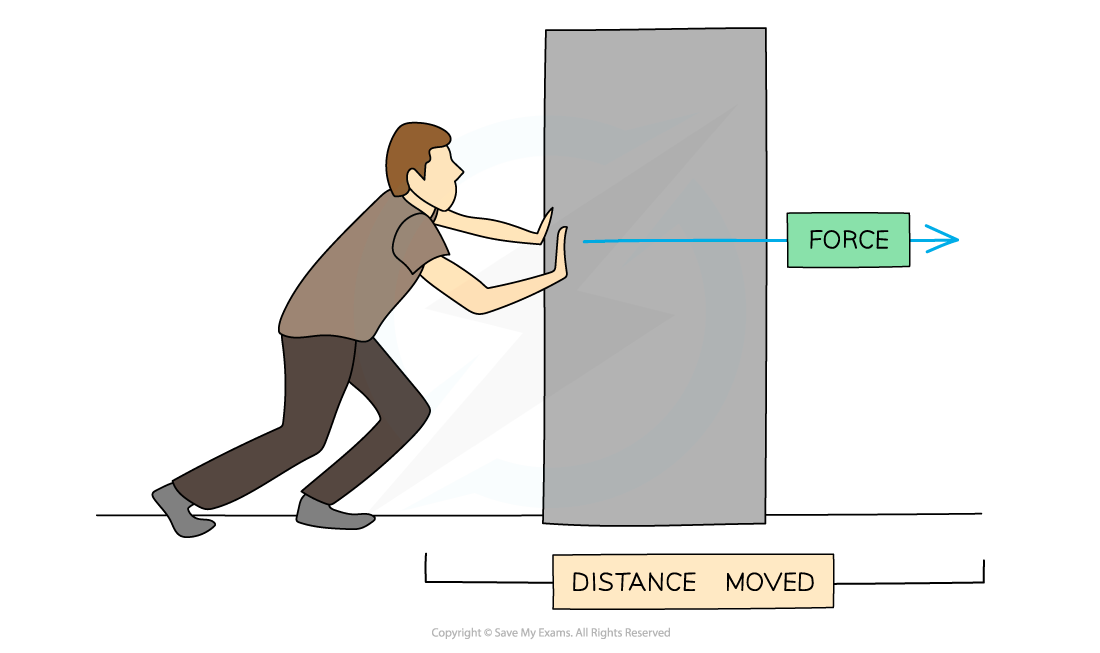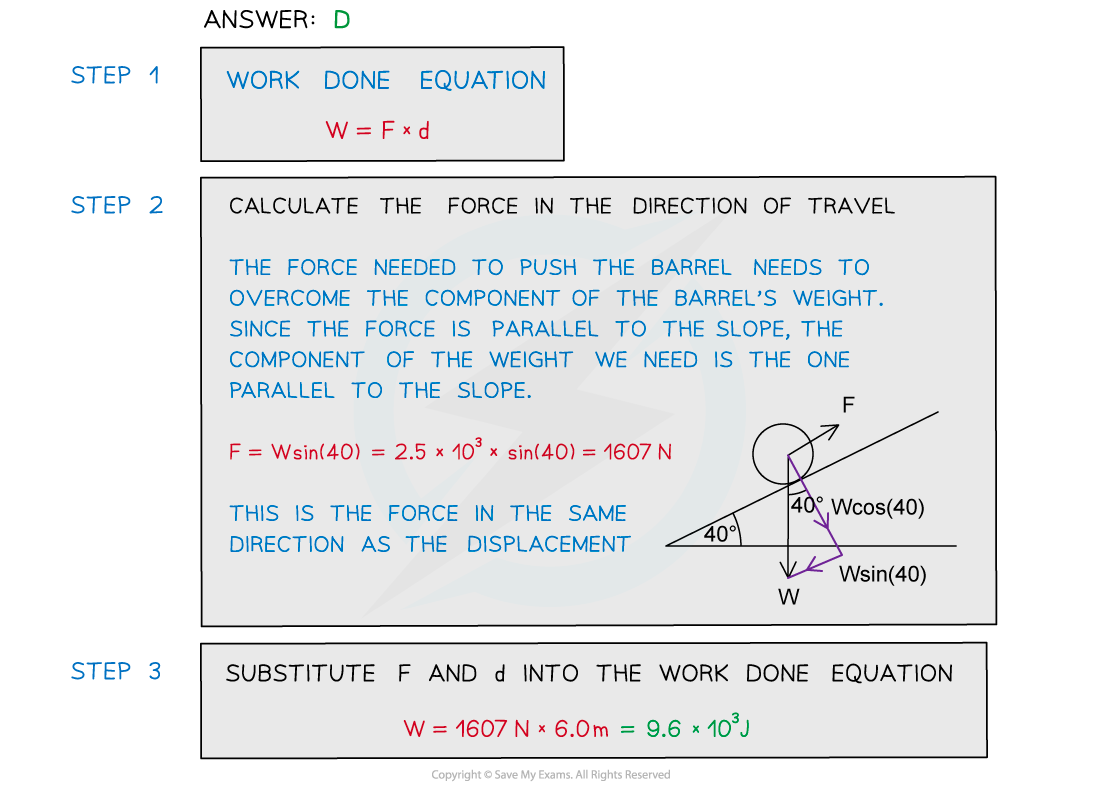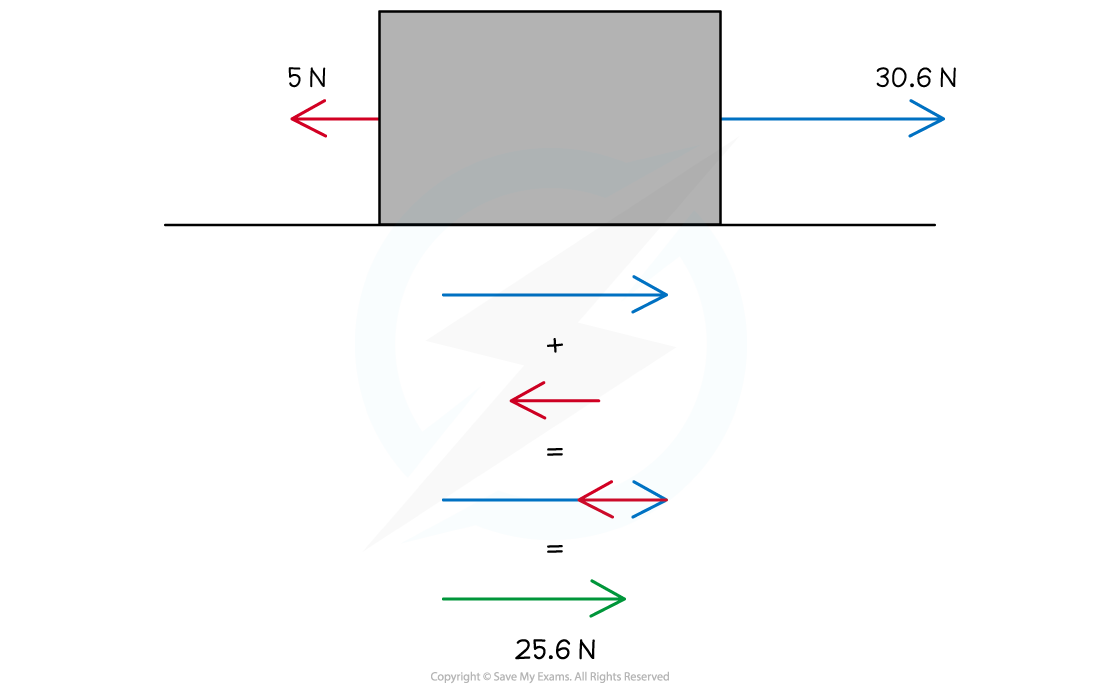# IB DP Physics: SL复习笔记2.3.4 Work Done

### Work Done

• Work is defined as

The amount of energy transferred when an external force causes an object to move over a certain distance

• If the force is parallel to the direction of the object's displacement, the work done can be calculated using the equation:

W = Fs

• Where:
• W = work done (J)
• F = average force applied (N)
• s = displacement (m)
• In the diagram below, the man’s pushing force on the block is doing work as it is transferring energy to the blockWork is done when a force is used to move an object over a distance

• When pushing a block, work is done against friction to give the box kinetic energy to move
• The kinetic energy is transferred to other forms of energy such as heat and sound
• Usually, if a force acts in the direction that an object is moving then the object will gain energy
• If the force acts in the opposite direction to the movement then the object will lose energy
• When plotting a graph of average force applied against displacement, the area under the graph is equal to the work done
• Sometimes the direction of motion of an object is not parallel to the direction of the force
• If the force is at an angle θ to the object's displacement, the work done is calculated by:

W = Fs cos θ

• Where θ is the angle, in degrees, between the direction of the force and the motion
• When θ is 0 (the force is in the direction of motion) then cos θ = 1 and W = Fs
• This may not always be cos θ, since this is just for horizontal motion
• For vertical motion, it would be sin θ
• Always consider the horizontal and vertical components of the force
• The component needed is the one that is parallel to the displacementWhen the force is at an angle, only the component of the force in the direction of motion is considered for the work done

#### Worked Example

The diagram shows a barrel of weight 2.5 × 103 N on a frictionless slope inclined at 40° to the horizontal.A force is applied to the barrel to move it up the slope at a constant speed. The force is parallel to the slope.What is the work done in moving the barrel a distance of 6.0 m up the slope?A.     7.2 × 103 J               B.     2.5 × 104 J              C.     1.1 × 104 J               D.     9.6 × 103 J#### Worked Example

An 80 kg person pulls a 15 kg box along using a rope which is at 40° from the horizontal as shown below. The person is pulling with a force of 40 N and moves the box 20 m horizontally from its starting position against a constant friction force of 5.0 N.Calculate the work that has been done on the box in the direction of its motion.Step 1: List the known quantities

• The angle between the rope and the horizontal, θ = 40°
• The pulling force (along rope) = 40 N
• Horizontal distance moved by box, s = 20 m
• Frictional force = 5.0 N

Step 2: Resolve the pulling force in the rope into its horizontal component• The horizontal component of the pulling force is the only part of the pulling force aligned with the direction of work
• Hence, that is the component that is needed to continue solving this problem
• The horizontal component can be resolved from:

cos(40°) × 40 = 30.6 N to the right

Step 3: Find the net force for the motion

• The net force can be found from the interaction between the horizontal component of the pulling force and the friction force:30.6 – 5.0 = 25.6 N to the right

Step 4: Use the equation for work

• Use the equation for work given the net force and distance moved in the horizontal

W = Fs cos θ

• The cos θ has already been accounted for so that the net force could be found when combining with friction
• Therefore:

W = F × s

W = 25.6 × 20 = 512 J

Step 5: State the final answer

• The work done on this box in the horizontal direction is:

W = 512 J

#### Exam Tip

Sometimes exam questions will include more values than you need to use in the solution - this is purposefully done to confuse you. For example, in the worked example above, the question supplies the mass of the person and the box, however, these quantities are not needed for the calculation.